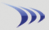Subscription & data-feed pricing Class schedule

Margin rates Stock & option commissions

Attention: Discussion forums are read-only for extended maintenance until further notice.

 Bullish Divergence Between RSI14 and Price Rate this Topic:Previous Topic · Next Topic Watch this topic · Print this topic ·
joandsig
 Posted : Monday, September 18, 2017 1:26:56 PMGold Customer

Joined: 6/13/2009
Posts: 5

Is there a way to scan for bullish divergence where RSI3 makes a higher low and at the same time price makes a lower low (Andrew cardwell). Let's say we want to do this for a 5 day period counting back from today.

It would be ideal if that scan could find such a constellation for different time frames as well but that same objective could also be met by using different scans (e.g. 6,7,8,9 days).

Thanks, Siggi

Al_Gorithm
 Posted : Monday, September 18, 2017 1:38:23 PMRegistered User
Joined: 6/30/2017
Posts: 1,227

Hi siggi,

you might get a faster response from the trainers in the Ask a Trainer forum. Sounds like it would be right up Bruce's alley.

Bruce_L
 Posted : Monday, September 18, 2017 1:55:59 PMWorden Trainer

Joined: 10/7/2004
Posts: 65,138

I'm not sure if you mean something simple like this:

`WRSI3 = MIN(WRSI3, 5) AND L > MINL5`

Which could be modified by just changing all of the 5s.

`WRSI3 = MIN(WRSI3, 6) AND L > MINL6`

And combined to check over multiple spans using OR:

`(WRSI3 = MIN(WRSI3, 5) AND L > MINL5) OR (WRSI3 = MIN(WRSI3, 6) AND L > MINL6) OR (WRSI3 = MIN(WRSI3, 7) AND L > MINL7) OR (WRSI3 = MIN(WRSI3, 8) AND L > MINL8) OR (WRSI3 = MIN(WRSI3, 9) AND L > MINL9)`

Or if you mean something more compicated where the low in WRSI is defined as having a higher value on each side, checking for this to have happened for the previous bar, finding when this happend most recently besides the last bar, and comparing the values of both WRSI an L at those two lows in WRSI (in the case of the following formula, that other low can have happened 22 bars ago at most).

`WRSI3 > WRSI3.1 AND WRSI3.1 < WRSI3.2 AND IIF(WRSI3.2 > WRSI3.3 AND WRSI3.3 < WRSI3.4, IIF(WRSI3.3 > WRSI3.1 AND L3 < L1, 3, 0), IIF(WRSI3.3 > WRSI3.4 AND WRSI3.4 < WRSI3.5, IIF(WRSI3.4 > WRSI3.1 AND L4 < L1, 4, 0), IIF(WRSI3.4 > WRSI3.5 AND WRSI3.5 < WRSI3.6, IIF(WRSI3.5 > WRSI3.1 AND L5 < L1, 5, 0), IIF(WRSI3.5 > WRSI3.6 AND WRSI3.6 < WRSI3.7, IIF(WRSI3.6 > WRSI3.1 AND L6 < L1, 6, 0), IIF(WRSI3.6 > WRSI3.7 AND WRSI3.7 < WRSI3.8, IIF(WRSI3.7 > WRSI3.1 AND L7 < L1, 7, 0), IIF(WRSI3.7 > WRSI3.8 AND WRSI3.8 < WRSI3.9, IIF(WRSI3.8 > WRSI3.1 AND L8 < L1, 8, 0), IIF(WRSI3.8 > WRSI3.9 AND WRSI3.9 < WRSI3.10, IIF(WRSI3.9 > WRSI3.1 AND L9 < L1, 9, 0), IIF(WRSI3.9 > WRSI3.10 AND WRSI3.10 < WRSI3.11, IIF(WRSI3.10 > WRSI3.1 AND L10 < L1, 10, 0), IIF(WRSI3.10 > WRSI3.11 AND WRSI3.11 < WRSI3.12, IIF(WRSI3.11 > WRSI3.1 AND L11 < L1, 11, 0), IIF(WRSI3.11 > WRSI3.12 AND WRSI3.12 < WRSI3.13, IIF(WRSI3.12 > WRSI3.1 AND L12 < L1, 12, 0), IIF(WRSI3.12 > WRSI3.13 AND WRSI3.13 < WRSI3.14, IIF(WRSI3.13 > WRSI3.1 AND L13 < L1, 13, 0), IIF(WRSI3.13 > WRSI3.14 AND WRSI3.14 < WRSI3.15, IIF(WRSI3.14 > WRSI3.1 AND L14 < L1, 14, 0), IIF(WRSI3.14 > WRSI3.15 AND WRSI3.15 < WRSI3.16, IIF(WRSI3.15 > WRSI3.1 AND L15 < L1, 15, 0), IIF(WRSI3.15 > WRSI3.16 AND WRSI3.16 < WRSI3.17, IIF(WRSI3.16 > WRSI3.1 AND L16 < L1, 16, 0), IIF(WRSI3.16 > WRSI3.17 AND WRSI3.17 < WRSI3.18, IIF(WRSI3.17 > WRSI3.1 AND L17 < L1, 17, 0), IIF(WRSI3.17 > WRSI3.18 AND WRSI3.18 < WRSI3.19, IIF(WRSI3.18 > WRSI3.1 AND L18 < L1, 18, 0), IIF(WRSI3.18 > WRSI3.19 AND WRSI3.19 < WRSI3.20, IIF(WRSI3.19 > WRSI3.1 AND L19 < L1, 19, 0), IIF(WRSI3.19 > WRSI3.20 AND WRSI3.20 < WRSI3.21, IIF(WRSI3.20 > WRSI3.1 AND L20 < L1, 20, 0), IIF(WRSI3.20 > WRSI3.21 AND WRSI3.21 < WRSI3.22, IIF(WRSI3.21 > WRSI3.1 AND L21 < L1, 21, 0), IIF(WRSI3.21 > WRSI3.22 AND WRSI3.22 < WRSI3.23, IIF(WRSI3.22 > WRSI3.1 AND L22 < L1, 22, 0), 0)))))))))))))))))))) > 0`

-Bruce
Personal Criteria Formulas
TC2000 Support Articles
Users browsing this topic
Guest-1

 Forum Jump Customer Training & Support - Ask a Trainer - TC2000 version 12/18 - Ask a Trainer - TC2000 version 7 - Ask a Trainer - StockFinder 5.0 - PCFs, EasyScan and Custom Indicators General Discussions - Stock and Market Talk - TC2000 version 12 or 18 - TC2000 version 7 - StockFinder 5.0 - RealCode for StockFinder 5.0 Tutorial Videos - TC2000 version 12 tutorial videos - TC2000 version 7 tutorial videos You cannot post new topics in this forum. You cannot reply to topics in this forum. You cannot delete your posts in this forum. You cannot edit your posts in this forum. You cannot create polls in this forum. You cannot vote in polls in this forum.